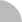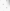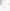(3.230.76.48) 您好！臺灣時間：2021/04/12 16:07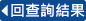### 詳目顯示:::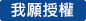: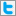Twitter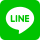•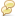被引用:2
•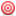點閱:468
•評分: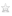•下載:9
•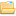書目收藏:0
 本論文為了彌補基本解法在物理域外人工邊界的缺陷，引入兩種改良方法，即邊界配置法及正規化無網格法；這兩種數值方法不僅可以將觀測點及源點放置於真實邊界，也可以保有基本解法的精神。我們使用誤差評估技術評估兩種方法的數值誤差，藉由觀察其誤差曲線，我們可獲得最佳配置點的數目；於採取最佳配置點後，在無解析解的情況下，該方法收斂的數值解可以被獲得。此外，我們藉由多重座標系統的概念改良這個誤差評估技術，解決本誤差評估技術於求解內域及外域多孔洞問題。最後，我們舉一些數值算例來驗證誤差評估技術的準確性和穩定性；本論文將多個散射體的水波問題置於最後來驗證此誤差評估技術的應用性及可行性。
 In this thesis, two improved approach, called the boundary collocation method (BCM) and the regularized meshless method (RMM) were introduced to remedy the drawback of the controversial artificial boundary outside the physical domain of the method of fundamental solutions (MFS). Not only can both numerical methods retain the spirit of the MFS, but they can also distribute the observation and the source points on the coincident locations of the real boundary. Furthermore, we implemented a proposed error estimation technique to estimate the numerical error of the two methods. By observing the obtained error curve, we derived the optimal number of collocation points. After adopting the optimal number of collocation points, the convergent solution of problem can be obtained in unavailable analytical solution condition. In addition, we improved this error technique by the concept of the multiply coordinate systems when we treat with the interior and exterior problem with multiply holes. Finally, we took the numerical examples to verify the accuracy and stability of the proposed error estimation technique. The water wave problem with multiply scatters was taken in the final numerical example to verify the applicable and practicable of this error technique.
 Contents ITable captions IVFigure captions VNotation captions XVIAbstract XX摘要 XXIChapter 1 Introduction1.1 Motivation of the research 11.2 Organization of the thesis 3Chapter 2 The method of fundamental solutions2.1 Introduction 42.2 Problems statement 52.2.1 Boundary value problem 52.2.2 Green’s function problem 62.2.3 Water wave problem 72.3 Formulation 92.4 The proposed error estimation technique 122.4.1 Definition of an auxiliary problem 122.4.1.1 Defining the G.E., contour and B.C. type 122.4.1.2 Giving the exact solution 132.4.1.3 Specifying B.C.s 142.4.1.4 Difference between the auxiliary problem and the original problem 142.4.2 Error analysis in the auxiliary problem 152.4.3 Solving the original problem 162.5 Illustrative examples and discussions 162.6 Conclusions 23Chapter 3 The boundary collocation method3.1 Introduction 253.2 Problems statement 263.3 Formulation 263.3.1 Meshless formulation using radial basis function of the imaginary-part kernel 273.3.2 Calculation for the diagonal elements in the four influence matrices using the rule and invariant method 283.4 The proposed error estimation technique 293.5 Illustrative examples and discussions 293.6 Conclusions 33Chapter 4 The regularized meshless method4.1 Introduction 354.2 Problems statement 364.3 Formulation 364.3.1 Meshless formulation using radial basis functions 364.3.2 Derivation of diagonal coefficients of influence matrices 384.3.2.1 Diagonal coefficients of interior type 384.3.2.2 Diagonal coefficients of exterior type 394.4 The proposed error estimation technique 434.5 Illustrative examples and discussions 444.6 Conclusions 49Chapter 5 Conclusions and further research5.1 Conclusions 505.2 Further research 51ReferencesTable 2-1 The complementary solution for the Helmholtz equation 62Fig. 1-1 The frame of this thesis. 63Fig. 1-2 History of the proposed error estimation technique. 64Fig. 2-1 The problem statement of boundary value problem, (a) the interior domain with multiply holes, (b) the exterior domain with multiply holes. 65Fig. 2-2 The problem statement of Green’s function problem, (a) the interior domain with multiply holes, (b) the exterior domain with multiply holes. 66Fig. 2-3 The problem statement of water wave problem with multiply holes, (a) x-z plane, (b) x-y plane. 67Fig. 2-4 The sketch of the concept of the multiply coordinate systems. 68Fig. 2-5 The flowchart of the formulation in implementing the proposed error estimation technique for MFS. 69Fig. 2-6 Problem sketch of the case 1 of example 2-1. 70Fig. 2-7 The error analysis versus the parameter d, for case 1 of example 2-1, (a) Single-layer potential approach, (b) Double-layer potential approach. 71Fig. 2-8 R.M.S error versus the number of collocation points for case 1 of example 2-1, (a) Single-layer potential approach, (b) Double-layer potential approach. 72Fig. 2-9 The field solution of original problem for case 1 of example 2-1, (a) Single-layer potential approach, (b) Double-layer potential approach. 73Fig. 2-10 Problem sketch of the case 2 of example 2-1. 74Fig. 2-11 The error analysis versus the parameter d, for case 2 of example 2-1, (a) Single-layer potential approach, (b) Double-layer potential approach. 75Fig. 2-12 R.M.S error versus the number of collocation points for case 2 of example 2-1, (a) Single-layer potential approach, (b) Double-layer potential approach. 76Fig. 2-13 The field solution of original problem for case 2 of example 2-1, (a) Single-layer potential approach, (b) Double-layer potential approach. 77Fig. 2-14 Problem sketch of the example 2-2. 78Fig. 2-15 The error analysis versus the parameter d, for example 2-2, (a) Single-layer potential approach, (b) Double-layer potential approach. 79Fig. 2-16 R.M.S error versus the number of collocation points for example 2-2, (a) Single-layer potential approach, (b) Double-layer potential approach. 80Fig. 2-17 The field solution of original problem for example 2-2, (a) Single-layer potential approach, (b) Double-layer potential approach. 81Fig. 2-18 Problem sketch of the example 2-3. 82Fig. 2-19 The error analysis versus the parameter d, for example 2-3, (a) Single-layer potential approach, (b) Double-layer potential approach. 83Fig. 2-20 R.M.S error versus the number of collocation points for example 2-3, (a) Single-layer potential approach, (b) Double-layer potential approach. 84Fig. 2-21 The field solution of original problem for example 2-3, (a) Single-layer potential approach, (b) Double-layer potential approach. 85Fig. 2-22 Problem sketch of the example 2-4. 86Fig. 2-23 The error analysis versus the parameter d, for example 2-4, (a) Single-layer potential approach, (b) Double-layer potential approach. 87Fig. 2-24 R.M.S error versus the number of collocation points for example 2-4, (a) Single-layer potential approach, (b) Double-layer potential approach. 88Fig. 2-25 The field solution of original problem for example 2-4, (a) Single-layer potential approach, (b) Double-layer potential approach. 89Fig. 2-26 Problem sketch of the case 1 of example 2-5. (Lx=Ly=1, x0=y0=0.8) 90Fig. 2-27 The error analysis versus the parameter d, for case 1 of example 2-5, (a) Single-layer potential approach, (b) Double-layer potential approach. 91Fig. 2-28 R.M.S error versus the number of collocation points for case 1 of example 2-5, (a) Single-layer potential approach, (b) Double-layer potential approach. 92Fig. 2-29 The field solution of original problem for case 1 of example 2-5, (a) Actual solution, (b) Single-layer potential approach, (c) Double-layer potential approach. 93Fig. 2-30 Problem sketch of the case 2 of example 2-5. (Lx=Ly=1, x0=y0=0.8) 94Fig. 2-31 The error analysis versus the parameter d, for case 2 of example 2-5, (a) Single-layer potential approach, (b) Double-layer potential approach. 95Fig. 2-32 R.M.S error versus the number of collocation points for case 2 of example 2-5, (a) Single-layer potential approach, (b) Double-layer potential approach. 96Fig. 2-33 The field solution of original problem for case 2 of example 2-5, (a) FEM, (a) Single-layer potential approach, (b) Double-layer potential approach. 97Fig. 2-34 Problem sketch of case 1 of example 2-6. (r=1, θinc=0°) 98Fig. 2-35 The error analysis versus the parameter d, of case 1 of example 2-6, (a) Single-layer potential approach, (b) Double-layer potential approach. 99Fig. 2-36 R.M.S error versus the number of collocation points of case 1 of example 2-6, (a) Single-layer potential approach, (b) Double-layer potential approach. 100Fig. 2-37 The field solution of original problem of case 1 of example 2-6, (a) Single-layer potential approach, (b) Double-layer potential approach. 101Fig. 2-38 Free surface elevation around cylinder of case 1 of example 2-6. 102Fig. 2-39 Problem sketch of case 2 of example 2-6. (r/l=1/3, θinc=0°) 103Fig. 2-40 The error analysis versus the parameter d, of case 2 of example 2-6, (a) Single-layer potential approach, (b) Double-layer potential approach. 104Fig. 2-41 R.M.S error versus the number of collocation points of case 2 of example 2-6, (a) Single-layer potential approach, (b) Double-layer potential approach. 105Fig. 2-42 The field solution of original problem of case 2 of example 2-6, (a) Single-layer potential approach, (b) Double-layer potential approach. 106Fig. 2-43 Free surface elevation around cylinder (1) and (2) of case 2 of example 2-6. 107Fig. 2-44 The resultant forces on the corresponding cylinder for case 2 of example 2-6, (a) Single-layer potential approach, (b) Double-layer potential approach. 108Fig. 2-45 Problem sketch of the case 1 of case 3 of example 2-6. (r/l=0.8) 109Fig. 2-46 The error analysis versus the parameter d, for case 3(a) of example 2-6, (a) Single-layer potential approach, (b) Double-layer potential approach. 110Fig. 2-47 R.M.S error versus the number of collocation points for case 3(a) of example 2-6, (a) Single-layer potential approach, (b) Double-layer potential approach. 111Fig. 2-48 The field solution of original problem for case 3(a) of example 2-6, (a) Single-layer potential approach, (b) Double-layer potential approach. 112Fig. 2-49 Free surface elevation around cylinder (1), (2), (3) and (4) for case 3(a) of example 2-6. 113Fig. 2-50 The resultant force on the corresponding cylinder for case 3(a) of example 2-6, (a) D. V. Evans (1997), (b) Single-layer potential approach, (c) Double-layer potential approach. 114Fig. 2-51 The error analysis versus the parameter d, for case 3(b) of example 2-6, (a) Single-layer potential approach, (b) Double-layer potential approach. 115Fig. 2-52 R.M.S error versus the number of collocation points for case 3(b) of example 2-6, (a) Single-layer potential approach, (b) Double-layer potential approach. 116Fig. 2-53 The field solution of original problem for case 3(b) of example 2-6, (a) Single-layer potential approach, (b) Double-layer potential approach. 117Fig. 2-54 Free surface elevation around cylinder (1), (2), (3) and (4) for case 3(b) of example 2-6. 118Fig. 2-55 The resultant force on the corresponding cylinder for case 3(b) of example 2-6, (a) Single-layer potential approach, (b) Double-layer potential approach. 119Fig. 3-1 The flowchart of the formulation in implementing the proposed error estimation technique for BCM. 120Fig. 3-2 The error analysis versus the number of collocation point for case 1 of example 3-1. 121Fig. 3-3 The convergence rate of the error analysis versus and computational time, (a) Single-layer potential approach, (b) Double-layer potential approach. 122Fig. 3-4 The field solution of original problem for case 1 of example 3-1, (a) Single-layer potential approach, (b) Double-layer potential approach. 123Fig. 3-5 The error analysis versus the number of collocation point for case 2 of example 3-1, (a) Single-layer potential approach, (b) Double-layer potential approach. 124Fig. 3-6 The convergence rate of the error analysis versus and computational time, (a) Single-layer potential approach, (b) Double-layer potential approach. 125Fig. 3-7 The field solution of original problem for case 2 of example 3-1, (a) Single-layer potential approach, (b) Double-layer potential approach. 126Fig. 3-8 The error analysis versus the number of collocation point for example 3-2, (a) Single-layer potential approach, (b) Double-layer potential approach. 127Fig. 3-9 The convergence rate of the error analysis versus and computational time, (a) Single-layer potential approach, (b) Double-layer potential approach. 128Fig. 3-10 The field solution of original problem for example 3-2, (a) Single-layer potential approach, (b) Double-layer potential approach. 129Fig. 3-11 The error analysis versus the number of collocation point for example 3-3, (a) Single-layer potential approach, (b) Double-layer potential approach. 130Fig. 3-12 The convergence rate of the error analysis versus and computational time, (a) Single-layer potential approach, (b) Double-layer potential approach. 131Fig. 3-13 The field solution of original problem for example 3-3, (a) Single-layer potential approach, (b) Double-layer potential approach. 132Fig. 3-14 The error analysis versus the number of collocation point for example 3-4, (a) Single-layer potential approach, (b) Double-layer potential approach. 133Fig. 3-15 The convergence rate of the error analysis versus and computational time, (a) Single-layer potential approach, (b) Double-layer potential approach. 134Fig. 3-16 The field solution of original problem for example 3-4, (a) Single-layer potential approach, (b) Double-layer potential approach. 135Fig. 3-17 The error analysis versus the number of collocation point for case 1 of example 3-5, (a) Single-layer potential approach, (b) Double-layer potential approach. 136Fig. 3-18 The convergence rate of the error analysis versus and computational time, (a) Single-layer potential approach, (b) Double-layer potential approach. 137Fig. 3-19 The field solution of original problem for case 1 of example 3-5, (a) Actual solution, (b) Single-layer potential approach, (c) Double-layer potential approach. 138Fig. 3-20 The error analysis versus the number of collocation point for case 2 of example 3-5, (a) Single-layer potential approach, (b) Double-layer potential approach. 139Fig. 3-21 The convergence rate of the error analysis versus and computational time, (a) Single-layer potential approach, (b) Double-layer potential approach. 140Fig. 3-22 The field solution of original problem for case 2 of example 3-5, (a) FEM, (b) Single-layer potential approach, (c) Double-layer potential approach. 141Fig. 4-1 The flowchart of the formulation in implementing the proposed error estimation technique for RMM. 142Fig. 4-2 The convergence rate of the error analysis versus and computational time for case 1 of example 4-1. 143Fig. 4-3 The field solution of original problem for case 1 of example 4-1. 144Fig. 4-4 The convergence rate of the error analysis versus and computational time for case 2 of example 4-1. 145Fig. 4-5 The field solution of original problem for case 2 of example 4-1. 146Fig. 4-6 The convergence rate of the error analysis versus and computational time for example 4-2. 147Fig. 4-7 The field solution of original problem for example 4-2. 148Fig. 4-8 The convergence rate of the error analysis versus and computational time for example 4-3. 149Fig. 4-9 The field solution of original problem for example 4-3. 150Fig. 4-10 The convergence rate of the error analysis versus and computational time for example 4-4. 151Fig. 4-11 The field solution of original problem for example 4-4. 152Fig. 4-12 The convergence rate of the error analysis versus and computational time for case 1 of example 4-5. 153Fig. 4-13 The field solution of original problem for case 1 of example 4-5. 154Fig. 4-14 The convergence rate of the error analysis versus and computational time for case 2 of example 4-5. 155Fig. 4-15 The field solution of original problem for case 2 of example 4-5. 156Fig. 4-16 The convergence rate of the error analysis versus and computational time for case 1 of example 4-6. 157Fig. 4-17 The field solution of original problem for case 1 of example 4-6. 158Fig. 4-18 Free surface elevation around cylinder of case 1 of example 4-6. 159Fig. 4-19 The convergence rate of the error analysis versus and computational time for case 2 of example 4-6. 160Fig. 4-20 The field solution of original problem for case 2 of example 4-6. 161Fig. 4-21 Free surface elevation around cylinder (1) and (2) of case 2 of example 4-6. 162Fig. 4-22 The resultant forces on the corresponding cylinder for case 2 of example 4-6. 163Fig. 4-23 The convergence rate of the error analysis versus and computational time for case 3(a) of example 4-6. 164Fig. 4-24 Free surface elevation around cylinder (1), (2), (3) and (4) for case 3(a) of example 4-6. 165Fig. 4-25 Free surface elevation around cylinder (1), (2), (3) and (4) for case 3(a) of example 4-6. 166Fig. 4-26 The resultant force on the corresponding cylinder for case 3(a) of example 4-6, (a) D. V. Evans (1997), (b) RMM. 167Fig. 4-27 The convergence rate of the error analysis versus and computational time for case 3(b) of example 4-6. 168Fig. 4-28 The field solution of original problem for case 3(b) of example 4-6. 169Fig. 4-29 Free surface elevation around cylinder (1), (2), (3) and (4) for case 3(b) of example 4-6. 170Fig. 4-30 The resultant force on the corresponding cylinder for case 3(b) of example 4-6. 171
 A. H. D. Cheng, "Particular solutions of Laplacian, Helmholtz-type, and polyharmonic operators involving higher order radial basis functions," Eng Anal Bound Elem vol. 24, pp. 531-538, 2000.A. H. D. Cheng, D. L. YoungL, and C. C. Tsai, "The solution of Poisson’s equation by iterative DRBEM using compactly supported, positive definite radial basis function," Eng Anal Bound Elem, vol. 24, pp. 549-575, 2000.A. N. Williams and W. Li, "Water wave interaction with an array of bottom-mounted surface-piercing porous cylinders," Ocean Eng, vol. 27, pp. 841-866, 2000.A. Poullikkas, A. Karageorghis, and G. Georgiou, "Methods of fundamental solutions for harmonic and biharmonic boundary value problems," Comput Mech vol. 21, pp. 416-423, 1998.B. H. Spring and P. L. Monkmeyer, "Interaction of plane waves with vertical cylinders," Proceeding of 14th International Conference on Coastal Engineering, pp. 1828-1845, 1974.B. Jin and W. Chen, "Boundary knot method based on geodesic distance for anisotropic problems," J Comput Phys, vol. 215, pp. 614-629, 2006.C. C. Tsai, D. L. Young, C. W. Chen, and C. M. Fan, "The method of fundamental solutions for eigenproblems in domains with and without interior holes," Proc R Soc A, vol. 462, pp. 1443-1466, 2006.C. F. Liao, "Applications of new error estimation technique in torsion and anti-plane shear problems with multiple inclusions " Master, National Ilan University, Ilan, Taiwan, 2012.C. M. Linton and D. V. Evans, "The interaction of waves with arrays of vertical circular cylinders," J Fluid Mech, pp. 215, 549-569, 1990.D. L. Young, K. H. Chen, and C. W. Lee, "Novel meshless method for solving the potential problems with arbitrary domains," Journal of Computational Physics, vol. 209, pp. 290-321, 2005.D. L. Young, K. H. Chen, and C. W. Lee, "Singular meshless method using double layer potentials for exterior acoustics," Journal of the Acoustical Society of America vol. 119, pp. 96-107, 2006.D. V. Evans and R. Porter, "Near-trapping of waves by circular arrays of vertical cylinders," Appl Ocean Res, vol. 19, pp. 83-99, 1997.D. V. Evans and R. Porter, "Trapping and near-trapping by arrays of cylinders in waves," J Eng Math, vol. 35, pp. 149-179, 1999.F. L. Jhong, "Applications of new error estimation technique to the method of fundamental solutions and Trefftz method for solving the boundary value problems of Helmholtz equation " Master, National Ilan University, Ilan, Taiwan, 2011.G. Duclos and A. H. Clement, "Wave propagation through arrays of unevenly spaced vertical piles," Ocean Eng, vol. 31, pp. 1655-1668, 2004.G. Fairweather and A. Karageorghis, "The method of fundamental solutions for elliptic boundary value problems," Adv Comput Math vol. 9, pp. 69-95, 1998.G. R. Liu and Y. T. Gu, "Meshless local Petrov-Galerkin (MLPG) method in combination with finite element approaches," Computational Mechanics, vol. 26, pp. 536-546, 2000.C. W. Hon YC, "Boundary knot method for 2D and 3D Helmholtz and convection–diffusion problems under complicated geometry," Int J Numer Methods Eng, 2003.I. Babuska and T. Strouboulis, The finite element method and its reliability Oxford: Oxford Univ, 2001.J. Li, H. Liu, and S. K. Tan, "Numerical study of wave trapping within cylinderical arrays," International Offshore and Polar Engineers Conference, 2012.J. P. Chen, C. X. Huang, and K. H. Chen, "Determination of spurious eigenvalues and multiplicities of true eigenvalues using the real-part dual BEM," Computational Mechanics, vol. 24(1), 41-51, 1999.J. Sladek, V. Sladek, and S. N. Atluri, "Local boundary integral equation (LBIE) method for solving problems of elasticity with nonhomogeneous material properties," Computational Mechanics, vol. 24, pp. 456-462, 2000.J. T. Chen, "An application of new error estimation technique in the meshless method and boundary element method," Master, National Ilan University, Ilan, Taiwan, 2010.J. T. Chen, I. L. Chen, K. H. Chen, Y. T. Lee, and Y. T. Yeh, "A meshless method for free vibration analysis of circular and rectangular clamped plates using radial basis function," Engineering Analysis with Boundary Elements, vol. 28, pp. 535-545, 2004.J. T. Chen and K. H. Hong, "Review of dual integral representations with emphasis on hypersingular integrals and divergent series," Transactions of the American Society of Mechanical Engineers, vol. 52, pp. 17-33, 1999.J. T. Chen, M. H. Chang, K. H. Chen, and I. L. Chen, "Boundary collocation method for acoustic eigenanalysis of three-dimensional cavities using radial basis function," Computational Mechanics, vol. 29, pp. 392-408, 2002.J. T. Chen, M. H. Chang, K. H. Chen, and S. R. Lin, "The boundary collocation method with meshless concept for acoustic eigenanalysis of two-dimensional cavities using radial basis function," J Sound Vib, vol. 257, pp. 667-711, 2002.J. T. Chen, S. K. Kao, W. M. Lee, and Y. T. Lee, "Eigensolutions of the Helmholtz equation for a multiply connected domain with circular boundaries using the multipole Trefftz method," Engineering Analysis with Boundary Elements, vol. 34, pp. 463-470, 2009.J. T. Chen, S. R. Kuo, K. H. Chen, and Y. C. Chenh, "Comment on “vibration analysis of arbitrary shaped membranes using non-dimensional dynamic influence function”," Journal of Sound and Vibration, vol. 235, pp. 156-171, 2000.J. T. Oden, "Adaptive modeling in solid mechanics," Second European Congress on Computational Mechanics, 2001.J. T. Oden and K. Vemaganti, "Estimation of local modeling error and goal-oriented modeling of heterogeneous materials; Part I: Error estimates and adaptive algorithms," J Comput Phys, vol. 164, 2000.J. T. Oden and S. Prudhomme, "Goal-oriented error estimation and adaptivity for the finite element method," Comput Math Appl, vol. 41, 2001.J. T. Oden and S. Prudhomme, "Estimation of modeling error in computational mechanics," J Comput Phys, vol. 182, pp. 496-515, 2002.J. T. Oden, S. Prudhomme, D. Hammerand, and M. Kuczma, "Modeling error and adaptivity in nonlinear continuum mechanics," Comput Methods Appl Mech Eng, vol. 190, p. 6663, 2001.J. T. Oden and T. I. Zohdi, "Analysis and adaptive modeling of highly heterogeneous elastic structures," Comput Methods Appl Mech Eng, vol. 148, p. 367, 1997.K. H. Chen, J. H. Kao, and J. T. Chen, "Regularized meshless method for antiplane piezoelectricity problems with multiple inclusions," CMC, vol. 9, pp. 253-279, 2009.K. H. Chen, J. H. Kao, J. T. Chen, D. L. Young, and M. C. Lu, "Regularized meshless method for multiply-connected-domain Laplace problems," Engineering Analysis with Boundary Elements, vol. 30, pp. 822-896, 2006.K. H. Chen, J. T. Chen, and J. H. Kao, "Regularized meshless method for solving acoustic eigenproblem with multiply-connected domain," Computer Modeling in Engineering & Sciences, vol. 16, pp. 27-39, 2006.K. H. Chen, M. C. Lu, and H. M. Hsu, "Regularized meshless method analysis of the problem of obliquely incident water wave," Engineering Analysis with Boundary Elements, vol. 35, pp. 355-362, 2011.K. Vemaganti and J. T. Oden, "Estimation of local modeling error and goal-oriented adaptive modeling of heterogeneous materials. II. A computational environment for adaptive modeling of heterogeneous elastic solids," Comput Methods Appl Mech Eng, vol. 190, p. 6089, 2001.M. A. Tournour and N. Atalla, "Efficient evaluation of the acoustic radiation using multipole expansion.," Int J Numer Methods Eng, vol. 46, pp. 825-837, 1999.M. Abramowitz and I. A. Stegun, "Handbook of mathematical functions with formulation graphs and mathematical tablesdover," ed. New York, 1972.M. Ainsworth, "A posteriori error estimation for fully discrete hierarchic models of elliptic boundary value problems on thin domains," Numer Math, vol. 80, p. 325, 1998.M. Ainsworth and J. T. Oden, A posteriori error estimation in finite element analysis. New York: Wiley, 2000.M. G. Larson and T. J. Barth, "A posteriori error estimation for adaptive discontinuous Galerkin approximations of hyperbolic systems," Lect Notes Comput Sci Eng, vol. 11, p. 363, 2000.M. Paraschivoiu, J. Peraire, and A. T. Patera, "A posteriori finite element bounds for linear-functional outputs of elliptic partial differential equations," Comput Methods Appl Mech Eng, vol. 150, p. 289, 1997.O. Z. Mehdizadeh and M. Paraschivoiu, "Investigation of a two-dimensional spectral element method for Helmholtz's equation," J Comput Phys, vol. 189, pp. 111-129, 2003.P. Houston and B. Suli, "hp-adaptive discontinuous Galerkin finite element methods for first-order hyperbolic problems," SIAM J Sci Comput, vol. 23,, p. 1226, 2001.R. Becker and R. Rannacher, "A feedback approach to error control in finite elements methods: Basic analysis and examples," East-West J Numer Math, vol. 4, p. 237, 1996.R. C. Maccamy and R. A. Fuchs, "Wave force on piles: A diffraction theory, Technical Memorandum No. 69, US Army Coastal Engineering Research Center (formerly Beach Erosion Board)," 1954.R. Mclver and D. V. Evans, "Approximation of wave forces on cylinder arrays," Appl Ocean Res, vol. 6, pp. 101-107, 1984.R. Song and W. Chen, "An investigation on the regularized meshless method for irregular domain problems," CMES, vol. 42, pp. 59-70, 2009.S. N. Atluri, J. Sladek, V. Sladek, and T. Zhu, "The local boundary integral equation (LBIE) and it's meshless implementation for linear elasticity," Computational Mechanics, vol. 25, pp. 180-198, 2000.S. N. Atluri and T. L. Zhu, "The meshless local Petrov-Galerkin (MLPG) approach for solving problems in elasto-statics," Computational Mechanics, vol. 25, pp. 169-179, 2000.S. N. Atluri and T. L. Zhu, "New concept in meshless methods," Int. J. Numer. Meth. Engng., vol. 47, pp. 537-556, 2000.S. N. Atluri and T. Zhu, "A new meshless local Petrov-Galerkin (MLPG) approach in computational mechanics," Computational Mechanics, vol. 22, pp. 117-127, 1998.S. Prudhomme and J. T. Oden, "On goal-oriented error estimation for elliptic problems: Application to the control of pointwise errors," Comput Methods Appl Mech Eng, vol. 176, p. 313, 1999.S. W. Kang, "Free vibration analysis of arbitrarily shaped plates with a mixed boundary condition using non-dimensional dynamic influence function," Journal of Sound and Vibration, vol. 256, pp. 533-549, 2002.S. W. Kang, I. S. Kim, and J. M. Lee, "Free Vibration Analysis of Arbitrarily Shaped Plates With Smoothly Varying Free Edges Using NDIF Method," Journal of Vibration and Acoustics, vol. 130, pp. 041010-1, 2008.S. W. Kang and J. M. Lee, "Application of free vibration analysis of membranes using the non-dimensional dynamic influence function," Journal of Sound and Vibration, vol. 234, pp. 455-, 2000.S. W. Kang and J. M. Lee, "Free vibration analysis of arbitrarily shaped plates with clamped edges using wave-type function," Journal of Sound and Vibration, vol. 242, pp. 9-26, 2001.S. W. Kang and J. M. Lee, "Free vibration analysis of arbitrarily shaped plates with clamped edges using wave-type functions," J Sound Vib, vol. 242, pp. 9-26, 2001.S. W. Kang, J. M. Lee, and Y. J. Kang, "Vibration analysis of arbitrarily shaped membranes using non-dimensional dynamic influence function," J Sound Vib, vol. 221, pp. 117-132, 1999.V. D. Kupradze and M. A. Aleksidze, "The method of functional equations for the approximate solution of certain boundary value problems," USSR Comput Math Math Phys, vol. 4, pp. 199-205, 1964.V. Sladek, J. Sladek, S. N. Atluri, and R. Van Keer, "Numerical integration of singularities in meshless implementation of local boundary integral equations," Computational Mechanics, vol. 25, pp. 394-403, 200.V. Twersky, "Multiple scattering of radiation by an arbitrary configuration of parallel cylinders," J Acoust Soc, vol. 24, pp. 42-46, 1952.W. Chen, "Symmetric boundary knot method," Eng Anal Bound Elem vol. 26, 2002.W. Chen, J. Lin, and F. Wang, "Regularized meshless method for nonhomogeneous problems," Engineering Analysis with Boundary Elements, vol. 35, pp. 253-257, 2011.W. Chen, J. Shi, and L. Chen, "Investigation on the spurious eigenvalues of vibration plates by non-dimensional dynamic influence function method," Engineering Analysis with Boundary Elements, vol. 33, pp. 885-889, 2009.W. Chen and M. Tanaka, "A meshfree integration-free and boundary-only RBF technique," Comput Math Appl, vol. 43, pp. 379-391, 2002.W. S. Hwang, L. P. Hung, and C. H. Ko, "Non-singular boundary integral formulations for plane interior potential problems," Int J Numer Methods Eng, vol. 53, pp. 1751-1762.Y. C. Hon and W. Chen, "Boundary knot method for 2D and 3D Helmholtz and the convection-diffusion problems with complicated geometry," Int J Numer Methods Eng, vol. 56, pp. 1931-1948, 2003.Y. H. Chen, "Wave-induced oscillations in harbors by permeable arc break-waters," Master, National Taiwan Ocean University, Keelung, Taiwan, 2004.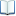電子全文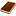國圖紙本論文推文當script無法執行時可按︰推文 網路書籤當script無法執行時可按︰網路書籤 推薦當script無法執行時可按︰推薦 評分當script無法執行時可按︰評分 引用網址當script無法執行時可按︰引用網址 轉寄當script無法執行時可按︰轉寄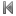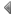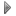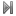top
 1 影響係數矩陣之對角線獲取技術應用於基本解法和正規化無網格法 2 以基本解法求解赫姆霍茲、擴散及柏格斯方程式 3 利用基本解法產生符合邊界的二維正交網格 4 Trefftz方法使用一般解求解3維Laplace方程 5 特解法結合基本解法中形狀參數變化的影響 6 正規化無網格法求解多連通邊界值問題 7 無網格法及邊界元素法於薄膜及板問題之退化尺度分析

 1 9. 翁順裕（2006），以交易成本經濟探討保險業之銀行通路－銀行保險，保險實務與制度，5（1），95-117。 2 7. 徐明松(1998)，「員工團體保險規劃實務」，空大學訊，第216期。 3 7. 徐明松(1998)，「員工團體保險規劃實務」，空大學訊，第216期。 4 5. 李瑞雲(1976)，團體保險基本型態的研究(一)，壽險季刊，第21期，頁9-15。 5 5. 李瑞雲(1976)，團體保險基本型態的研究(一)，壽險季刊，第21期，頁9-15。 6 9. 翁順裕（2006），以交易成本經濟探討保險業之銀行通路－銀行保險，保險實務與制度，5（1），95-117。 7 11. 陳武宗(1988)，「壽險行銷與管理的基本概念」，保險專刊 第14輯 8 11. 陳武宗(1988)，「壽險行銷與管理的基本概念」，保險專刊 第14輯

 1 新誤差評估技術應用於含夾雜之扭矩及反平面問題 2 影響係數矩陣之對角線獲取技術應用於基本解法和正規化無網格法 3 新誤差評估技術應用於基本解法和Trefftz方法求解赫姆赫茲邊界值問題 4 新誤差評估技術應用於基本解法和邊界元素法 5 正規化無網格法求解多連通邊界值問題 6 應用正規化無網格法探討含系列結構物的水波問題及其布拉格效應 7 煅燒頁岩灰應用於混凝土力學性質與耐久性之探討 8 土木結構試驗課程革新之研究 9 潛沒式導流板尾跡渦流及馬蹄渦之數值模擬及分析 10 深覆土隧道之冰凍工法暫態地溫場反算分析 11 基礎設施資產價值評估模式建構之研究－以國道橋梁為例 12 正規化概念分析應用於類別資料分類之研究 13 不同流況下丁壩群之間距對河岸保護及河道穩定效果之研究 14 利用自然通風設計改善室內熱環境之研究 -以國立宜蘭大學行政大樓為例 15 天然災害造成工期展延之調適方案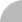簡易查詢 | 進階查詢 | 熱門排行 | 我的研究室